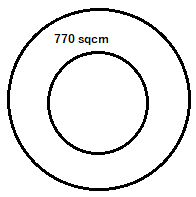mycollegehive
The area enclosed between two concentric circles is 770 sq cm. If the radius of outer circle is 21cm. Find the radius of the inner circle.
CBSE class-10 math areas-related-to-circles0The area enclosed between two concentric circles is 770 sq cm. If the radius of outer circle is 21cm. Find the radius of the inner circle.

19 viewsShareFollowUniversity of Lagos Nigeria
31 July 2020School not set Nigeria
31 July 20200Area of a circle = πr2

The area between the two circles is given as 770 sqcm.

If the radius of the outer circle is 21cm, it's area will be:

π(21)2 = 441π

Therefore, the area of the inner circle = 441π - 770

= 615.44 sqcm

Also, area = πr2

615.44 = πr2

divide both sides by π

r2 = 195.9

r = 14cm

Therefore, the radius of the inner circle is 14cmShare### Related Tags

CBSE

0 followers

56 questionsclass-10

0 followers

56 questionsmath

2 followers

985 questions